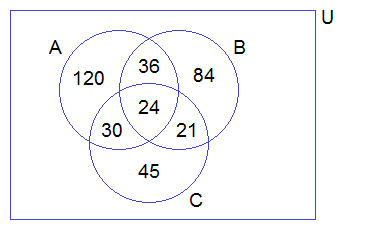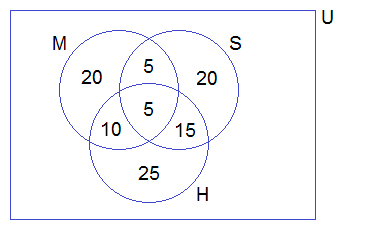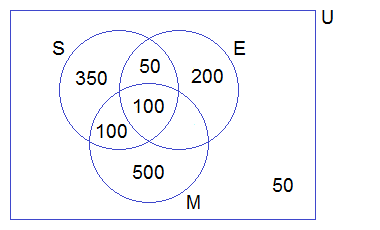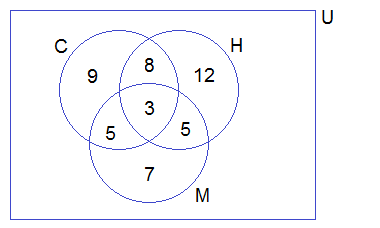## Cardinality Relations of Three Sets

Let, U be the universal set. A, B and C are three overlapping sets which are subsets of the universal set U.

********************

10 Math Problems officially announces the release of Quick Math Solver, an Android App on the Google Play Store for students around the world.

Look at the following Venn diagram and learn the cardinal representation for the different portions.n(A) = Number of elements in set A

n(B) = Number of elements in set B

n(C) = Number of elements in set C

no(A) = Number of elements in set A only

no(B) = Number of elements in set B only

no(C) = Number of elements in set C only

n(A∩B) = Number of elements common in set A and B

n(A∩C) = Number of elements common in set A and C

n(B∩C) = Number of elements common in set B and C

no(A∩B) = Number of elements common in set A and B only

no(A∩C) = Number of elements common in set A and C only

no(B∩C) = Number of elements common in set B and C only

n(A∩B∩C) = Number of elements common to A, B and C

n(A∪B∪C) = Number of elements belongs to at least one set A, B or C.

n(A∩B∩C)c = Number of elements does not belong to the set A, B or C.

### Formula on Cardinality Relations of Three Sets

If A, B and C are subsets of universal set U then,

1. n(A∪B∪C) = n(A) + n(B) + n(C) – n(A∩B) – n(B∩C) – n(A∩C) + n(A∩B∩C)

2. n(A∩B∩C) = n(A∪B∪C) – n(A) – n(B) – n(C) + n(A∩B) + n(B∩C) + n(A∩C)

3. n(A∪B∪C)c = n(U) – n(A∪B∪C)

4. no(A) = n(A) – n(A∩B) – n(A∩C) + n(A∩B∩C)

5. no(B) = n(B) – n(A∩B) – n(B∩C) + n(A∩B∩C)

6. no(C) = n(C) – n(A∩C) – n(B∩C) + n(A∩B∩C)

7. no(A∩B) = n(A∩B) – n(A∩B∩C)

8. no(B∩C) = n(B∩C) – n(A∩B∩C)

9. no(A∩C) = n(A∩C) – n(A∩B∩C)

10. Exactly two = no(A∩B) + no(B∩C) + no(A∩C)

11. Exactly one = no(A) + no(B) + no(C)

12. At least one = n(A∪B∪C)

13. All three = n(A∩B∩C)

14. None of them = n(A∪B∪C)c

### Word Problems Based on Three Sets

#### Workout Examples

Example 1: P, Q and R are subsets of a universal set U. If n(U) = 390, n(P) = 210, n(Q) = 165, n(R) = 120, n(P∩Q) = 60, n(Q∩R) = 45, n(A∩R) = 54 and n(P∩Q∩R) = 24, illustrate this information in a Venn-diagram and find the following:

a. no(P)

b. no(Q)

c. no(R)

d. no(P∩Q)

e. no(Q∩R)

f. no(P∩R)

g. n(P∪Q∪R)c

Solution:

Here,

Venn-diagram,From the Venn-diagram above,

a. no(P) = 120

b. no(Q) = 84

c. no(R) = 45

d. no(P∩Q) = 36

e. no(Q∩R) = 21

f. no(P∩R) = 30

g. n(P∪Q∪R)c = 30

Example 2: In a survey of a group of people, 60 liked tea, 45 liked coffee, 30 liked milk, 25 liked coffee as well as tea, 20 liked tea as well as milk, 15 liked coffee as well as milk and 10 liked all three. How many people were asked this question? Solve by using Venn-diagram.

Solution:

Let C, T and M represent the set of people who liked coffee, tea and milk respectively. Then,

∴ n(T) = 60

n(C) = 45

n(M) = 30

n(C∩T) = 25

n(T∩M) = 20

n(C∩M) = 15

n(C∩T∩M) = 10

Venn diagram,Now,

n(T∪C∪M)

= n(T) + n(C) + n(M) – n(C∩T) – n(T∩M) – n(C∩M) + n(C∩T∩M)

= 60 + 45 + 30 – 25 – 20 – 15 + 10

= 85

Hence, the required number of people = 85.

Example 3: In an examination, 40% of candidates passed in mathematics, 45% in Science, and 55% in Health. If 10% passed in Mathematics and Science, 20% in Science and Health and 15% in Health and Mathematics,

(i) Illustrate the above information by drawing a Venn diagram.

(ii) Find the pass percentage in all three subjects.

Solution:

Let M, S, and H denote the set of students who passed in Maths, Science and Health respectively.

∴ n(U) = 100% = n(M∪S∪H)

n(M) = 40%

n(S) = 45%

n(H) = 55%

n(M∩S) = 10%

n(S∩H) = 20%

n(H∩M) = 15%

Since, there is no one who failed in all three subjects,

n(M∪S∪H) = n(U) = 100%

(i) Venn-diagram,(ii) n (M∩S∩H)

= n(M∪S∪H) – n(M) – n(S) – n(H) + n(M∩S) + n(S∩H) + n(M∩H)

= 100 – 40 – 45 – 55 + 10 + 20 + 15%

= 5%

∴ 5% passed in all three subjects.

Example 4: Out of 1350 candidates, 600 passed in Science, 700 in Mathematics, 350 in English and 50 failed in all three subjects. If 200 passed in Science and Mathematics, 150 in Science and English, 100 in Mathematics and English,

(i) How many candidates passed in all three subjects?

(ii) Illustrate the above information in a Venn-diagram.

Solution:

Let, the set of candidates who passed in Science, Mathematics and English be S, M, and E.

∴ n(U) = 1350

n(S) = 600

n(M) = 700

n(E) = 350

n(S∪M∪E)c = 50

n(S∩M) = 200

n(M∩E) = 100

n(S∩E) = 150

We know,

n(S∪M∪E)

= n(U) – n(S∪M∪E)c

= 1350 – 50

= 1300

Now,

n(A∩B∩C)

= n(A∪B∪C) – n(A) – n(B) – n(C) + n(A∩B) + n(B∩C) + n(A∩C)

= 1300 – 600 – 700 – 350 + 200 + 100 + 150

= 100

Therefore,

(i) 100 candidates passed in all three subjects.

(ii) Venn-diagram,Example 5: In a group of students, 25 study computer, 28 study Health, 20 study Mathematics, 9 study Computer only, 12 study Health only, 8 study Computer and Health only and 5 students study Health and Mathematics only.

(i) Draw a Venn diagram to illustrate the above information.

(ii) Find how many students study all the subjects.

(iii) How many students are there altogether?

Solution:

Let C, H, and M be the set of students who study Computer, Health, and Mathematics respectively.

∴ n(C) = 25

n(H) = 28

n(M) = 20

no(C) = 9

no(H) = 12

no(C∩H) = 8

no(H∩M) = 5

Filling the above information in Venn-diagram, we getFrom the above Venn-diagram,

n(C∩H∩M) + 8 + 12 + 5 = n(H)

or, n(C∩H∩M) + 25 = 28

or, n(C∩H∩M) = 28 – 25

or, n(C∩H∩M) = 3

Again,

no(C∩M) + n(C∩H∩M) + 9 + 8 = n(C)

or, no(C∩M) + 3 + 9 + 8 = 25

or, no(C∩M) + 20 = 25

or, no(C∩M) = 25 – 20

or, no(C∩M) = 5

Again,

no(M) + no(C∩M) + n(C∩H∩M) + 5 = n(M)

or, no(M) + 5 + 3 + 5 = 20

or, no(M) + 13 = 20

or, no(M) = 20 – 13

or, no(M) = 7

(i) Venn-diagram:(ii) n(C∩H∩M) = 3 students study all subjects.

(iii) From the above venn-diagram,

n(C∪H∪M)

= 9 + 8 + 3 + 5 + 12 + 5 + 7

= 49

∴ There are 49 students altogether.

Example 6: In a group of people, 20 like milk, 30 like tea, 22 like coffee, 12 like coffee only, 6 like milk and coffee only, 2 like tea and coffee only and 8 like milk and tea only. Show these information in a Venn-diagram and find:

(i) How many like at least one drink?

(ii) How many like exactly one drink?

Solution:

Let the set of people who like milk, tea, and coffee be M, T, and C.

∴ n(M) = 20

n(T) = 30

n(C) = 22

no(C) = 12

no(M∩C) = 6

no(T∩C) = 2

no(M∩T) = 8

Filling the above information in the Venn-diagram, we getFrom the above Venn-diagram,

n(M∩T∩C) + 6 + 12 + 2 = n(C)

i.e. n(M∩T∩C) + 20 = 22

or, n(M∩T∩C) = 22 – 20

or, n(M∩T∩C) = 2

Again,

no(M) + 6 + n(M∩T∩C) + 8 = n(M)

i.e. no(M) + 6 + 2 + 8 = 20

or, no(M) + 16 = 20

or, no(M) = 20 – 16

or, no(M) = 4

Again,

no(T) + 2 + n(M∩T∩C) + 8 = n(T)

i.e. no(T) + 2 + 2 + 8 = 30

or, no(T) + 12 = 30

or, no(T) = 30 – 12

or, no(T) = 18

Venn-diagram,From the venn-diagram,

(i) n(M∪C∪T)

= 4 + 6 + 12 + 2 + 18 + 8 + 2

= 52

∴ 52 like at least one drink.

(ii) no(M) + no(C) + no(T)

= 4 + 12 +18

= 34

∴ 34 like exactly one drink.

If you have any questions or problems regarding the Word Problems Based on Three Sets, you can ask here, in the comment section below.